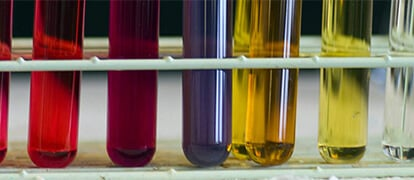# Chemical Reactions Year 12 Chemistry Students Must Know

A helpful list of essential chemical reactions that a Year 12 student should know.## Combustion of a Carbon Compound

Hydrocarbon + Oxygen → Carbon Dioxide Gas + Liquid Water

E.g. 2C8H18(l) + 25O2(g) → 16CO2(g) + 18H2O(l)

## Metals with Acids

Metal + Acid → Salt + Hydrogen gas

E.g. Zn(s) + 2HCl(aq) → ZnCl2(aq) + H2(g)

## Carbonates with Acids

Carbonate + Acid → Salt + Carbon Dioxide Gas + Liquid Water

E.g. Na2CO3(aq) + 2HCl(aq) → 2NaCl(aq) + CO2(g) + H2O(l)

## Oxidation

Metal → Metal Ion + Electron/s

E.g. Cu(s) → Cu2+(aq) + 2e

## Reduction

Metal Ion + Electron/s → Metal

E.g. Ag+(aq) + e → Ag(s)

## Redox (Reduction-Oxidation Reactions)

This reaction involves the transfer of electron/s from one reactant to another. This thus results in both a reduction and oxidation reaction occurring. To obtain the redox reaction, you can either write the net ionic equation for a displacement reaction (option 1) or add reduction and oxidation reactions together (option 2).

E.g. OPTION 1:

Cu(s) + 2AgNO3(aq) → Cu(NO3)2(aq) + 2Ag(s)

Cu(s) + 2Ag+(aq) + 2NO3 → Cu2+(aq) + 2NO3 + 2Ag(s)

Cu(s) + 2Ag+(aq) + 2NO3 → Cu2+(aq) + 2NO3 + 2Ag(s)

Cu(s) + 2Ag+(aq) → Cu2+(aq) + 2Ag(s)

E.g. OPTION 2

NOTE: BOTH THE CONSERVATION OF MASS AND CHARGE MUST BE SHOWN IN YOUR EQUATION!

Cu(s) → Cu2+(aq) + 2e

+

2Ag+(aq) + 2e → 2Ag(s)

__________________

Cu(s) + 2Ag+(aq) + 2e → Cu2+(aq) + 2e + 2Ag(s)

Cu(s) + 2Ag+(aq) → Cu2+(aq) + 2Ag(s)

## Lime Water Test (Lime Water and Carbon Dioxide)

Calcium hydroxide + Gaseous carbon dioxide  Solid calcium carbonate + liquid water

I.e. Ca(OH)2(aq) + CO2(g) → CaCO3(s) + H2O(l)

## Hydrogenation

Alkene + Hydrogen → Alkane

E.g. C2H4(g) + H2(g) → C2H6(g)                                           Catalyst is Pt(s)

## Bromination

Alkene + Bromine → Vicinal dibromide

E.g. C2H4(g) + Br2(g) → CH2BrCH2Br(g)

## Chlorination

Alkene + Chlorine → Vicinal dichloride

E.g. C2H4(g) + H2(g) → CH2ClCH2Cl(g)

## Hydrohalogenation

Alkene + HX → Alkyl halide

E.g. C2H4(g) + HBr(g) → CH3CH2Br(g)

## Substitution with Bromine

Alkene + Bromine → Organobromine compound + Hydrogen bromide

E.g. C2H6(g) +Br2(l) → C2H5Br(l) +HBr(g)

NOTE: This reaction requires the presence of UV light.

## Hydration

Alkene + Water → Alkanol

E.g. C2H4(g) + H2O(g) → CH3CH2OH(g)                             Catalyst is dilute H2SO4

## Dehydration

Alkanol → Alkene + Water

E.g. CH3CH2OH(g) → C2H4(g) + H2O(g)                             Catalyst is concentrate H2SO4

## Fermentation of Glucose

Aqueous Glucose → Ethanol + Carbon Dioxide Gas

I.e. C6H12O6(aq) → 2CH3CH2OH(aq) + 2CO2(g)                  Catalyst is zymase (found in yeast)

## Alpha Decay

Parent nucleus → Daughter nucleus + Alpha particle

E.g.  238U → 234Th + 4He

## Beta Decay

Parent nucleus → Daughter nucleus + Beta particle

E.g. 14C → 14N + e

## Neutralisation

Acid + Base → Salt + Liquid Water

E.g. HCl(aq) + NaOH(aq) → NaCl(aq) + H2O(l)

## Carbon Dioxide and Water

Aqueous carbon dioxide + Liquid water ↔ Carbonic Acid

I.e. CO2(aq) + H2O(l)  H2CO3(aq)

## Formation of Sulfur Dioxide

Sulfur + Gaseous oxygen → Sulfur Dioxide

I.e. S(s) + O2(g) → SO2(g)

## Formation of Sulfurous Acid

Gaseous sulfur dioxide + Liquid water → Sulfurous acid

I.e. SO2(g) + H2O(l)  H2SO3(aq)

## Formation of Sulfur Trioxide

Gaseous sulfur dioxide + Gaseous oxygen → Sulfur trioxide

I.e. 2SO2(g) + O2(g) → 2SO3(g)

## Formation of Sulfuric Acid

Gaseous sulfuric acid + Liquid Water → Sulfuric acid

I.e. SO3(g) + H2O(l) → H2SO4(aq)

## Formation of Nitrogen Monoxide

Gaseous oxygen + Gaseous nitrogen → Gaseous nitrogen monoxide

I.e. O2(g) + N2(g) → 2NO(g)

## Formation of Nitrogen Dioxide

Gaseous nitrogen monoxide + Gaseous oxygen → Gaseous nitrogen dioxide

I.e. 2NO(g)  + O2(g) → 2NO2(g)

## Formation of Nitric Acid

Gaseous nitrogen dioxide + Liquid water → Nitric acid + Gaseous nitrogen monoxide

I.e. 3NO2(g) + H2O(l) → 2HNO3(aq) + NO(g)

## Ionisation of an Acid in Water

Acid + Liquid Water → Hydronium Ion + Anion

E.g. HCl(aq) + H2O(l) → H3O+(aq) + Cl(aq)

E.g. H2SO4(aq) + H2O(l) → H3O+(aq) + HSO4(aq)

## Esterification

Ethanoic acid reacts with ethanol in the presence of concentrated sulphuric acid as a catalyst to produce the ester, ethyl ethanoate. The reaction is slow and reversible. To reduce the chances of the reverse reaction happening, the ester is distilled off as soon as it is formed.

Alkanoic acid + Alcohol  Alkyl alkanoate + Water               (Catalyst: Concentrated sulfuric acid)

E.g. CH3COOH(aq) + CH3CH2OH(aq)  CH3COOCH2CH3(aq) + H2O(l)

## Formation of Ozone

1. Gaseous oxygen + High energy UV → Oxygen free radical

I.e. O2(g) + UV → 2O(g)

2. Gaseous oxygen + Oxygen free radical → Gaseous ozone

I.e. O2(g) + O(g) → O3(g)

## Destruction of Ozone

1. Photodissociation of CFC

E.g. CF2Cl2(g) + UV → CF2Cl + Cl

2. Destruction of Ozone

I.e. O3(g) + Cl(g) → ClO(g) + O2(g)

3. Regeneration of Chlorine

I.e. ClO(g)+ O(g) → Cl(g) + O2(g)

## Are you struggling to stay on top of Chemistry?

Our HSC experts will make sure you know all the essential chemical reactions and more to Ace Year 12 Chemistry. Learn more!### Get free study tips and resources delivered to your inbox.

Join 75,893 students who already have a head start.

Our website uses cookies to provide you with a better browsing experience. If you continue to use this site, you consent to our use of cookies. Read our cookies statement.